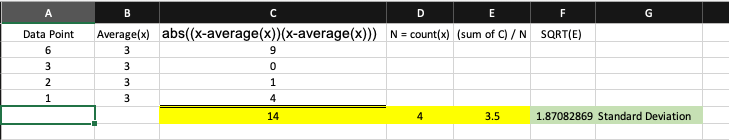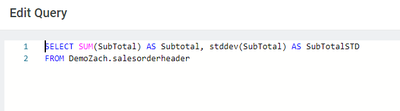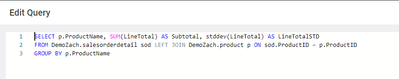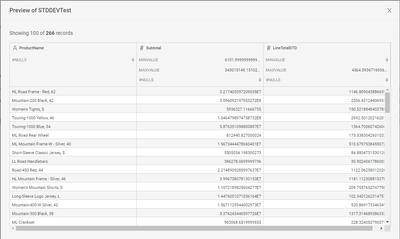cancel
Showing results for
Did you mean:

## Standard DeviationPartner

Hi,

=IFERROR(STDEV.P(BQ14348:CB14348)/AVERAGE(BQ14348:CB14348),0)

Could please let me know how to convert above "STDEV.P" excel function in Incorta.

3 REPLIES 3Employee

KHK,

Unfortunately, no we do not have a built in function for this.  We have many of the functions that in aggregate support the calculation such as ABS(), AVERAGE() and SQRT(). In a multi pass process it would look something like:

1. for each data point x calculate distance from mean, squared: abs((x-avg(x))(x-avg(x)))
2. sum each of the above, and divide by count(x)
3. sqrt(#2)

However, the easier way to do this is by using an python MV and using a python dataframe function to calculate the STDEV from the elements in the dataframe.  Pandas supports something like

`df['amount'].std()`EmployeeEmployee

Adding on to Jeffs approach, if you happen to be more SQL oriented you can alternatively use SparkSQL or Postgres SQL 'STDDEV' Function within a Materialized View.Output:Similarly, if we want to show the standard deviation grouped by product in the same scenario here is a simple example.

With Group By:Output:Regards,

Zach